• import scipy.io as io import cv2 mat_dir=r'D:\research\ce_Faster-RCNN-TensorFlow-Python3-master\data\VOCdevkit2007\VOC2007\mat...print('a is:',a['data'])#打印mat文件字典中的值 print('a.shape',a['data'].s
import scipy.io as io
import cv2
mat_dir=r'D:\research\ce_Faster-RCNN-TensorFlow-Python3-master\data\VOCdevkit2007\VOC2007\mat-JPEGImaes'#mat路径
print('a is:',a['data'])#打印mat文件字典中的值
print('a.shape',a['data'].shape)#查看数组形状
cv2.imwrite('./0.bmp',a['data'])#保存为图片
#print(a.keys())  #查看变量名字，发现是x，y，z
image_dir='./JPEGImages'

print('img_b',b)
print('img_b_shape',b.shape)

print('c:',c)

if (b==c).any():
print('true')

展开全文• Matlab读取DCM文件保存为NII或者NPZ或者MAT https://blog.csdn.net/tianjinyikedaxue/article/details/114870499
• 最近在做图像分割需要制作样本，Matlab自带的ImageLabeler工具就能方便地进行样本的标记，不过它只能将输出的样本数据保存为mat文件，为了方便在其他地方也能轻松使用这些样本，需要把mat文件转化为图片进行保存。...

最近在做图像分割需要制作样本，Matlab自带的ImageLabeler工具就能方便地进行样本的标记，不过它只能将输出的样本数据保存为mat文件，为了方便在其他地方也能轻松使用这些样本，需要把mat文件转化为图片进行保存。
对于低版本的matlab，下面这个博客已经给出了很详细的说明https://blog.csdn.net/tsyccnh/article/details/50812632，然而我使用的是Matlab2018a中的ImageLabeler工具，与之前低版本的工具相比做了一定的改动，查了一些资料后终于操作成功，下面就详细讲述下心得。

1.制作样本并输出为mat文件

在Matlab的APP工具中打开Image Labeler点击New Session新建一个会话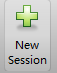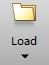加载图像后，点击Define New ROI Label新建一个类别可进行样本标记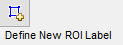填写该类别的名称，选择Rectangle则以矩形框的方式制作训练样本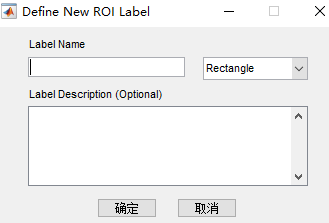另外还需要点击Export Labels把制作的样本保存为mat文件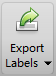2.将mat文件转换为图片保存

在试验中我共选取了两个类别的样本，名称为building何notbuilding，输出的label_2_classes_train.mat文件保存在当前目录下，接下来就具体分析如何使用mat文件。
首先加载mat文件：

可以看到显示的变量名称为gTruth，类型为groundTruth对象。通过gTruth.LabelData返回存放样本数据的table。

gTruth.LabelData

output:

1×2 table
building notbuilding
_____________ _____________
[28×4 double] [30×4 double]

这个table的大小为1x2，通过它的Properties操作可以获取table的一些属性。例如要获取table中变量的名称：

gTruth.LabelData.Properties.VariableNames

output:

1×2 cell 数组
{‘building’} {‘notbuilding’}

我们要获取table中的元素，可以用{}进行索引操作：

gTruth.LabelData{1,1}

output:

1×1 cell 数组
{28×4 double}

返回的是gTruth.LabelData中的第1行第1列的元素，类型为cell数组，这个cell数组中的元素为一个28x4的double型数组。我们要获取这个cell中的元素，可以用{}进行索引操作。

gTruth.LabelData{1,1}{1,1}

output:

ans =
353 25 14 12
560 16 12 9

返回的数组中的每一行表示一个矩阵框选样本的位置(xmin,ymin,width,height)，这个与Imcrop(img,rect)中的rect中的格式一致。
获取一个样本的位置操作为：

gTruth.LabelData{1,1}{1,1}(1,:)

output:

ans =
353 25 14 12

这样一来我们就成功的获取了所需要的数据。

下面是将mat文件转化为图片的函数：

function SaveImagesFromImageLabeler(img_path,mat_filepath,save_dir)
%UNTITLED 将使用ImageLabeler标记的样本的mat文件转化为tiff格式的图片保存
%parameters:
%--img_path:
%meaning:用来制作样本的图像的路径
%type：string
%--mat_filepath:
%meaning:使用Export Labels输出的mat文件的路径，如‘1.mat’
%type：string
%--save_dir:
%meaning:保存图像的文件夹
%type：string
%%

table=gTruth.LabelData;
%获取table的变量名，即类别名称,返回一个cell
class_name=table.Properties.VariableNames;
%判断存放图像的文件夹是否存在，不存在则创建
n_class=size(class_name,2);
for i=1:n_class
savepath=fullfile(save_dir,class_name{1,i});
disp(savepath);
if exist(savepath,'dir')==0
mkdir(savepath);
end
end
%遍历table中的每一类别的cell
for i=1:n_class
%获得每一类中的样本图片数目
class_array=table{1,i}{1,1};
num_img=size(class_array,1);
%遍历每一类的所有图片
for j=1:num_img
img_sample=imcrop(img,class_array(j,:));
img_sample_savepath=fullfile(save_dir,class_name{1,i},[num2str(j),'.tiff']);
disp(img_sample_savepath);
imwrite(img_sample,img_sample_savepath);
end
end

end

主函数为：

%保存训练样本和测试样本图片

%%
%保存训练样本图片
train_img_path='1.tif';
train_mat_filepath='label_2_classes_train.mat';
train_save_dir='data\train';

SaveImagesFromImageLabeler(train_img_path,train_mat_filepath,train_save_dir);
disp('保存训练样本图片结束');

展开全文matlab 样本标注
• matlab图片批量预处理并保存为mat数据和jpg文件。~~预处理中的代码可以替换成其他方式，这里只是做个引导。批处理的文件夹以及图片的格式请根据实际情况自行调整。图像批处理
• 首先我们谈谈MarkDown编辑器，我感觉些倒是挺方便的，因为用惯了LaTeX，对于MarkDown还是比较容易上手的，但是我发现，MarkDown中有这样几个问题一直没能找到具体的解决方法：图片大小的问题。在LaTeX中我们可以调整...

首先我们谈谈MarkDown编辑器，我感觉些倒是挺方便的，因为用惯了LaTeX，对于MarkDown还是比较容易上手的，但是我发现，MarkDown中有这样几个问题一直没能找到具体的解决方法：

图片大小的问题。在LaTeX中我们可以调整图片的大小，以适应整个文本；

字体，字号大小的设置。在MarkDown里面标题倒是挺大的，但是正文却显得太小，不是很喜欢里面的字体。

主要发现上面两个问题导致编辑出来的文本挺难看。

一、mat文件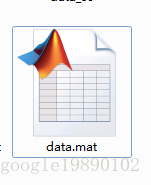save('data_1.mat','A')

其中，'A'表示要保存的内容。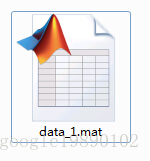二、python中读取mat文件

1、读取文件

如上例：

#coding:UTF-8

'''

Created on 2015年5月12日

@author: zhaozhiyong

'''

import scipy.io as scio

dataFile = 'E://data.mat'

注意，读取出来的data是字典格式，可以通过函数type(data)查看。

print type(data)

结果显示

找到mat文件中的矩阵：

print data['A']

结果显示

[[ 0. 0. 0. 0. 0. 0. 0.

0. 0. 0. 0. 0. 0. 0.

。。。。。。。。。。。

0. 0. 0. 0. 0. 0. 0.

0.36470588 0.90196078 0.99215686 0.99607843 0.99215686 0.99215686

0.78431373 0.0627451 0. 0. 0. 0. 0.

0. 0. 0. 0. 0. 0. 0.

。。。。。。。。。。。。

0.94117647 0.22745098 0. 0. 0. 0. 0.

0. 0. 0. 0. 0. 0. 0.

0. 0. 0. 0. 0. 0. 0.

0. 0. 0. 0. 0. 0.30196078

。。。。。。。

0. 0. 0. 0. 0. 0. 0.

0. 0. 0. 0. 0. 0. 0. ]]

格式为：

即为numpy中的矩阵格式。

2、保存文件

将这里的data['A']矩阵重新保存到一个新的文件dataNew.mat中：

dataNew = 'E://dataNew.mat'

scio.savemat(dataNew, {'A':data['A']})

注意：是以字典的形式保存。

展开全文• 单个 .mat 文件转换 import scipy.io as scio ...dataFile = r'./CoNSeP/Train/Labels/train_2.mat' # 单个的mat文件 data = scio.loadmat(dataFile) print(type(data)) # print (data['data']) # 由于导

单个 .mat 文件转换

import scipy.io as scio
from PIL import Image
import numpy as np
import matplotlib.pyplot as plt
import os

dataFile = r'./CoNSeP/Train/Labels/train_2.mat' # 单个的mat文件
print(type(data))
# print (data['data'])
# 由于导入的mat文件是structure类型的，所以需要取出需要的数据矩阵
a=data['inst_map']
# 取出需要的数据矩阵

# 数据矩阵转图片的函数
def MatrixToImage(data):
data = data*255
new_im = Image.fromarray(data.astype(np.uint8))
return new_im

new_im = MatrixToImage(a)
plt.imshow(a, cmap=plt.cm.gray, interpolation='nearest')
new_im.show()
new_im.save('train_2.png') # 保存图片

批处理

import scipy.io as scio
from PIL import Image
import numpy as np
import matplotlib.pyplot as plt
import os

data_dir = './CoNSeP/Train/Labels'
path = './CoNSeP/Train/Labels/'
img_ids    =   sorted(os.listdir(data_dir))
print(len(img_ids))

for img_id in img_ids:
dataFile =  path + img_id  # 单个的mat文件
print(type(data))
# print (data['data'])
# 由于导入的mat文件是structure类型的，所以需要取出需要的数据矩阵
a=data['inst_map']
# 取出需要的数据矩阵

# 数据矩阵转图片的函数
def MatrixToImage(data):
data = data*255
new_im = Image.fromarray(data.astype(np.uint8))
return new_im

new_im = MatrixToImage(a)
# plt.imshow(a, cmap=plt.cm.gray, interpolation='nearest')
# new_im.show()
new_im.save(img_id[:-4] + '.png') # 保存图片

展开全文python 机器学习
• % %读入图片保存为mat文件 clear all; close all; clc; %将图像的文件夹路径读入 %假设flowers文件夹下有许多flowers1.png,flowers2.png等等 namelist = dir(fullfile('F:\MATLAB2017b\AMOutputResults\flowers...MATLAB
• Mat img; fs ; // Read: FileStorage fs("img.xml", FileStorage::READ); Mat img; fs >> img; 解决方案 Writing to file cv::FileStorage storage("test.yml", cv::FileStorage::WRITE); storage ; storage....cv mat保存图片
• a=load('Pose29_64x64.mat'); %文件名 for i = 1:1 :3332 %数据循环 b = a.fea(i,:); %一行一行的读取 d = b/255;... %图片大小64*64 %imshow(D) sc=strcat('C:\Users\801\Desktop\555\',sp
• 目的：将100张图片保存为.mat文件，每一行是一张图片（一个cell），点进去是346*286的矩阵。 clc; clear all; filepath = 'F:\test_png\'; %图片路径可以根据自己需要修改; ext='*.png'; dis=dir([filepath ext]) ...matlab
• 用Clear all,clc,先把变量全清除之后load yourMatFileName.mat %yourMatFileName.mat为保存图像的mat文件 再用whos命令查看导入了什么变量，这些变量就存放着数据。再用imshow(Variable)，就可以查看图像了详细请看...
• matlab实现批量图片mat转换，对应大量数据集图片处理异常重要 clc; clear all; num = 1000; %图片数量 for i = 1 : num 　IMAGE = imread(sprintf('G:\\TEST\\%.jpg', i)); 　eval(sprintf('IMAGE%d = IMAGE;', ...
• 图片转为mat格式保存-matlab

千次阅读 2020-11-27 15:16:07
namelist = dir('D:\PythonProject\*.png'); #文件路径 file_name = {namelist...#用于重命名文件 批量保存 for i=1:l I = imread([dirs,file_name{i}]); savePath = [file_name{i},'.mat']; save(savePath,'I') endmatlab
• pyhton读取tif(高光谱)图片保存为mat文件 1.安装并导入所需要的库 scipy 和 skimage !pip install scipy !pip install scikit-image import scipy.io as sio import skimage.io 2.读入tif图 path = r'1.tif' ...python matlab
• 由于手中的数据是mat文件（我的数据是一组乳腺切片组成的三维数据），想要查看某一张切片的图片，又不想使用软件打开，因此需要利用脚本进行转换。 A = sio.loadmat(r'41582_L_MLO') B = A['imageCroped'] 首先读取...
• 但是我们也在提交测试时，也会需要提交.mat文件。下面我们就将得到的测试图片保存在四维数组中，并生成.mat的字典格式。 import numpy as np import os.path import shutil from scipy.io.matlab.mio import ...
• MATLAB实例：将批量的图片保存为.mat文件一、彩色图片 1. MATLAB程序clc; clear all;num = 17;for i = 1 : numIM = imread(sprintf('E:\\将批量的图片保存为mat\\horse\\horse%03d.jpg', i));eval(sprintf('IM%d = ...
• Matlab将图片存为.mat文件

万次阅读 2018-02-09 15:42:46
• 1. matlab批量修改文件名 比如文件夹里有很多 .nii 后缀的文件，需要统一修改成 clc; clear all; file = dir('*.nii'); 2. matlab批量读取文件夹内所有图片
• Python将npy文件转换为mat文件用到的模块有numpy，scipy，osimport numpy as npimport scipy.io as ioimport osdef npy_mat(npy_path,mat_path):npyname_path = os.listdir(npy_path)for npyname in npyname_path:...
• python-mat转为图片保存

千次阅读 2018-10-09 21:52:00
数据如上所示，主文件夹下有/home/kls/Desktop/data/ucf101_fea_video/51_v_Lungers_g21_c05.matmat第一维fea_tmp也是一个mat，第二维fea_spa也是一个mat，我们要将fea_tmp转换jpg import cv2 import scipy...
• 1. 打开需要保存的图 2. 运行语句： h1 = findobj(gcf,'Color','r') xh1=get(h1,'xdata'); yh1=get(h1,'ydata'); save time xh1; save Q-courese-sin-disturbance yh1; %%save 文件名 变量名； load Q-courese...MATLAB figure
• .mat文件批量转成图片——python脚本编写

万次阅读 热门讨论 2018-05-15 09:22:22
欢迎使用Markdown编辑器写博客 本Markdown编辑器使用...图片链接和图片上传 LaTex数学公式 UML序列图和流程图 离线写博客 导入导出Markdown文件 丰富的快捷键 快捷键 加粗 Ctrl + B 斜体 Ctrl + I...python脚本 .mat文件转换 批量操作
• Mnist数据集，matlab版本，可以直接读入，大小784，每个标签对应0~9大小，另外建议各位在使用时使用one-hot处理数据
• 图片与.mat文件的转换 使用Matlab对图片进行操作时，大量的单张图片不利于操作，此时需要将图片转化.mat格式，.mat方便我们对数据的处理，使我们更加高效的利用数据。实验中每张图片的名称是连续的数字，相同类别...
• MATLAB实现mat文件批处理转化png格式（全网仅有！） 由于部分算法（如边缘检测算法），跑出来的结果图是带有白色边框的，如下图：（跑过程序，做过算法的人都知道） 那么这后续处理提供了极大的不便，因为空白...matlab 人工智能 机器学习 python
• Python解析mat文件

千次阅读 2020-04-10 16:26:08
mat文件 mat文件是matlab的数据存储的标准格式。mat文件是标准的二进制文件,还可以ASCII...很多数据集的标签都是通过mat文件来存储的，为了解析出图片所对应的的标签信息，我们需要从mat文件中来解析出这些信息， ...mat文件...